# The Triangle Inequality

The Triangle Inequality
Go back to  'Triangles'

### What is the Triangle Inequality?

The Triangle Inequality (theorem) says that in any triangle, the sum of any two sides must be greater than the third side. For example, consider the following triangle, ∆ABC: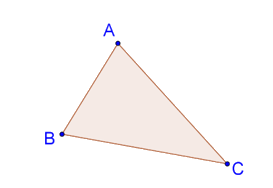According to the Triangle Inequality,

• AB + BC must be greater than AC, or AB + BC > AC.
• AB + AC must be greater than BC, or AB + AC > BC
• BC + AC must be greater than AB, or BC + AC > AB.

### Visualising the Triangle Inequality

Drag the vertices of ∆ABC below to vary it, and note how the Triangle Inequality holds true for each pair of sides of the triangle:

### Why is the Triangle Inequality true?

An easy way to understand why the Triangle Inequality works in any ∆ABC is to imagine yourself walking along the sides of the triangle. If you have to go from A to B, for example, the shortest path will be the segment AB. If you first go to C and then to B, the distance you cover, AC + CB, will surely be greater than AB.

Alternatively, let's try and understand the Triangle Inequality through a construction. Suppose that you are given three lengths: x, y and z. You are asked to construct a triangle with these sides. You proceed as follows:

1. First, you draw a segment AB of length z units.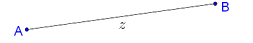2. Next, keeping the tip of your compass at A, you draw an arc of length x units.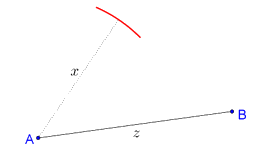3. Then, keeping the tip of your compass at B, you draw an arc of length y units, in a way so that it intersects the earlier arc.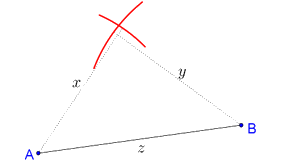4. The point of intersection is your third vertex C. You join A to C and B to C, and thus you have your triangle: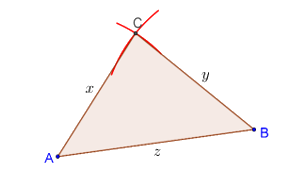The question now is: can this always be done? Suppose that the length y was so small that your second arc could never intersect your first arc (which had a radius of x units):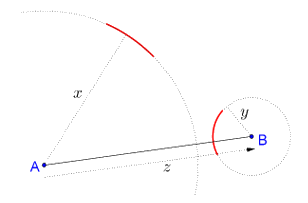In this case, a triangle cannot be formed with these three lengths. Observe carefully that the two arcs will intersect only if the sum of the radii of the two arcs is greater than the distance between the centres of the arc. In other words, to be able to draw a triangle:

x + y must be greater than z

This means, for example, that there can be no triangle with sides 2 units, 2 units and 5 units, because:

2 + 2 < 5

This is the basic idea behind the Triangle Inequality.

### Proof of the Triangle Inequality

Let us now discuss a proof of the Triangle Inequality. Consider the following triangle, ∆ABC:Let us prove that AB + AC > BC.

Proof: Extend BA to point D such that AD = AC, and join C to D, as shown below:We note that ∠ACD = ∠D, which means that in ∆BCD, ∠BCD > ∠D. Sides opposite larger angles are larger, and thus:

BD > BC

è AB + AD > BC

è AB + AC > BC (because AD = AC)

This completes our proof. We can additionally conclude that in a triangle, since the sum of any two sides is greater than the third, this means that the difference of any two sides will be less than the third. As an exercise, prove this statement rigorously.

More Important Topics
Numbers
Algebra
Geometry
Measurement
Money
Data
Trigonometry
Calculus